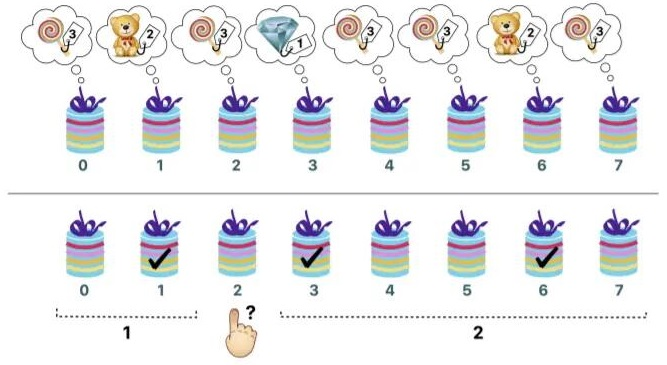# #323. 【IOI2017】The Big Prize

“大奖”是一个家喻户晓的TV游戏节目。这次你很幸运地进入到最后一轮。已知编号从 $0$ 到 $n-1$ 的 $n$ 个盒子从左到右排成一行，你就站在这排盒子的前面。每个盒子里面放有一个奖品，必须打开盒子才能看到是什么奖品。已知有 $v\ge 2$ 种不同类型的奖品。这 $v$ 种类型按照奖品价值的降序从 $1$ 到 $v$ 排列。

• 在 $i$ 号盒子左面的盒子中，刚好有 $a$ 个盒子中的奖品，价值比 $i$ 号盒子中的奖品价值要高。
• 在 $i$ 号盒子右面的盒子中，刚好有 $a$ 个盒子中的奖品，价值比 $i$ 号盒子中的奖品价值要高。

• $0$ 号盒子和 $1$ 号盒子中恰好有一个盒子中的奖品比 $2$ 号盒子中的奖品更贵。
• 在 $3,4,...,7$ 号盒子中恰好有 $2$ 个盒子中的奖品比 $2$ 号盒子中的奖品更贵。

### 实现细节

 int find_best(int n)
• 此函数只被评测程序调用仅一次。
• $n$: 盒子的数量.
• 你实现的这个函数应该返回装有钻石的盒子编号，即，唯一的整数 $d$（$0\le d\le n-1$）使得 $d$ 号盒子放有类型为 $1$ 的奖品。

 std::vector<int> ask(int i)
• $i$: 你在询问时选择的盒子编号。$i$ 的数值必须介于 $0$ 和 $n-1$ 之间（含）。
• 这个函数返回包含 $2$ 个元素的数组 $a$。其中，$a$ 是在 $i$ 号盒子的左面，奖品比 $i$ 号盒子的奖品价值更高的盒子数目。而 $a$ 则是在 $i$ 号盒子右面，奖品比 $i$ 号盒子的奖品价值更高的盒子数目。

### 例子

• ask(0) 返回 $[0,3]$
• ask(1) 返回 $[0,1]$
• ask(2) 返回 $[1,2]$
• ask(3) 返回 $[0,0]$
• ask(4) 返回 $[2,1]$
• ask(5) 返回 $[2,1]$
• ask(6) 返回 $[1,0]$
• ask(7) 返回 $[3,0]$### 数据范围

• $3\leq n\leq 200000$
• 每个盒子中奖品的类型介于 $1$ 和 $n$ 之间（含）。
• 类型 $1$ 的奖品恰有一个。
• 对于所有 $2\leq t\leq v$，如果类型 $t-1$ 的奖品有 $k$ 个，那么类型 $t$ 的奖品将严格多于 $k^2$ 个。

$1$ 恰好有 $1$ 个钻石和 $n-1$ 个棒棒糖（所以，$v=2$），你可以调用函数 ask 最多 $10000$ 次 $20$
$2$ 无附加限制 $80$

$10000 < q$ $0$（Wrong Answer
$6000 < q \leq 10000$ $70$
$5000 < q \leq 6000$ $80-(q-5000)/100$
$q\leq 5000$ $80$

### 评分程序样例

• 第 $1$ 行：$n$
• 第 $2$ 行：$p\ p\ \cdots\ p[n-1]$